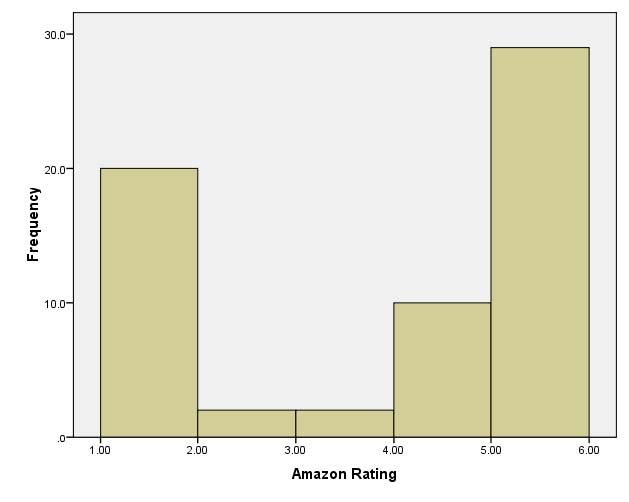# Multiple choice questions

Quizzes are available to test your understanding of the key concepts covered in each chapter. Click on the quiz below to get started.

1.    Below is a histogram of ratings of Britney Spears’s CD, Britney. What can we say about the data from this histogram?1. The data are normally distributed.
2. The median rating was 2.
3. The modal score is 5.
4. The data are leptokurtic.

The correct answer is c) The modal score is 5. This is because the tallest bar represents the mode, and this bar is for a rating of 5.

2.    In a small data sample (N = 20), what can we say about a z-score of 2.37?

1. It is significant at p < .05
2. It is significant at p < .001
3. It is significant at p < .01
4. It is non-significant

The correct answer is a) It is significant at p < .05. This is because an absolute value greater than 1.96 is significant at p < .05, above 2.58 is significant at p < .01 and above 3.29 is significant at p < .001